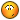## Recommended Posts

Hi All,

I'm trying to read all the rows and columns in Excel, if Row A2 is Y, my test will be executed, if N, test will get terminated and i have 10 tests (rows) in my data sheet.

I want to loop though each one of the row and check the value of A2 (this is static now, want to make it as dynamic) Y or N, i'm trying with the following code but since i'm hard coded A2, i'm unable to read A3, A4,A5 so on, Can you please help me on this?

Local \$sWorkbook = "Data.xlsx"
Local \$oExcel = _Excel_Open()
Local \$oWorkbook = _Excel_BookOpen(\$oExcel,\$sWorkbook)
Local \$iRows = \$oExcel.ActiveSheet.UsedRange.Rows.Count

For \$i = 2 To \$iRows Step 1
MsgBox(\$MB_SYSTEMMODAL, "", "Count down!" & @CRLF & A2)
If \$svalue = "Y" Then
MsgBox(\$MB_SYSTEMMODAL, "", "Value of A2" & @CRLF & \$svalue )

Else

Exit
EndIf

Next

##### Share on other sites

Hey,

You can use the \$i while reading the range:

```Local \$sWorkbook = "Data.xlsx"
Local \$oExcel = _Excel_Open()
Local \$oWorkbook = _Excel_BookOpen(\$oExcel, \$sWorkbook)
Local \$iRows = \$oExcel.ActiveSheet.UsedRange.Rows.Count

For \$i = 2 To \$iRows Step 1
Local \$svalue = _Excel_RangeRead(\$oWorkbook, "sheetName", "A"&\$i)
;~  MsgBox(\$MB_SYSTEMMODAL, "", "Count down!" & @CRLF & A2) ; What's this line for?
If \$svalue = "Y" Then
MsgBox(\$MB_SYSTEMMODAL, "", "Value of A"&\$i & @CRLF & \$svalue)
Else
Exit
EndIf
Next```

On a side note: you can read the whole range instead of line by line (I think that would run faster) and do what you want in that array (see https://www.autoitscript.com/autoit3/docs/libfunctions/_Excel_RangeRead.htm again):

```Local \$iRows = \$oExcel.ActiveSheet.UsedRange.Rows.Count

##### Share on other sites

Fantastic, this is working fine, thanks for your prompt response.

But when i give the range "A2:A or A:A ", getting the value of the row as Zero.

```Local \$iRows = \$oExcel.ActiveSheet.UsedRange.Rows.Count

##### Share on other sites

That's because if you put a range of cells the return value is an array.

Quote

## Return Value

 Success: the data from the specified cell(s). A string for a cell, a zero-based array for a range of cells.

##### Share on other sites

Got it, Thank you so much:-)

##### Share on other sites

You're welcome## Create an account

Register a new account

• ### Recently Browsing   0 members

×

• Wiki

• Back

• #### Beta

• Git
• FAQ
• Our Picks
×
• Create New...